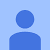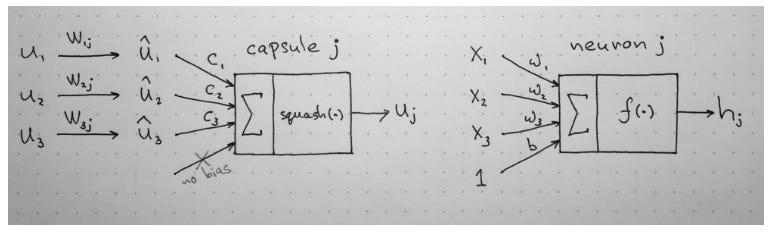# Intuition of Capsule in CapsNet Clearly Explained

Source: Deep Learning on Medium

Intuition of Capsule in CapsNet Clearly Explained

What you will be learning in the article.

• What is capsule?
• What is advantage of CapNet over CNN?
• Intuitive understanding of how does CapNet and capsule work?
• How do human brains use Inverse Graphic Approach to understand 3D worlds?
• How does Dynamic Routing Between Capsule work?
• Examples of capsules factor analysis

Terminology to remember: Capsule means vector.

### Intuition of Capsule what what it does?

Capsule is an idea to that intent to capture properties of input dataset in vector instead of scalar which is commonly used in CNN and other popular Deep Learning Architecture. In the case of CapNet, it deals with images input.

Why vectors? vectors has 2 properties: orientation (relationship) and length (probability that capsule entity exists) while scalar only have one, value. Using vectors to capture properties, we can make assumption such as the following:

There must be relationship between output vector of one layer and output vector of another layer if causes output vector of one causes output vector of another layer to rotate in the same direction

Another advantage of using vector instead of scalar is that it allows the network to captures more than 1 properties of input. For each of the captures properties, using technique, introduce by Geoffrey Hinton, called “Dynamic Routing Between Capsule,” it allows the network to construct hierarchical structure of all captured properties.

To quickly summarize what I mentioned above, scalar only allows you to ask

“how likely do these features exist in the given input”

while vector allows you to ask 2 questions:

“1. How likely do these features exists in this picture

2. What is the relationship of this features with respect to other features of the given input?”

Given the above picture, CNN may think that the one of the right is a face, it just does not show in the input dataset, so CNN will predict no. On the other hand, CapsNet think that the picture on the right cannot be a face, because position of eye relative to mouth, nose, and face does not resemble a face, so CapsNet will predict no. As you can see, both model predict the answer correctly but they answer different questions before generating answers.

If you have problem visualizing, lets me give you example to help you visualize.

### How do human brains use Inverse Graphic Approach to understand 3D worlds?

This is an argument made by Geoffrey Hinton that human brain approach ot understanding 3D world. Computer Graphics graphics construct 3D images by stacking internal hierarchical representation of geometric data. It represent geometric data by using relative position of the object.

That internal representation is stored in computer’s memory as arrays of geometrical objects and matrices that represent relative positions and orientation of these objects.

This approach is different from view point invariant CNN utilizes to classify images.

Pooling layer, for example, sum of value of nearby center pixel. This function resulted in view point invariant. As mention by Geoffrey Hinton, view point invariant is sensitive to quality and quantity of data.

Imaging there is video of a face turning to one side, frame by frame you would start to see one of the eyes disappear. and the face is completely turned CNN will have to find pattern in dataset to see that there exist a face with 1 eye. (turned face)

This is different from CapNets in that CapNets is capable of extrapolate by infinite amount of affine transformation which means that once the shape of a face and its part are recognized, turning face would be recognize as a face + rotation to the side in vector format. because of this capability of CapNets, view of above, left, right, and scale up/down of the same object will be alot easier to learn. However, objects can be stretch, and bend will be harder to recognized because these are non-linear transformation.

We perform mental rotatoin to decide if the tilted R has the correcet handedness, not to recognized that it is a R.

if you are like me, you will have to rotate R first before you realize that R have incorrect rotation. This is because we recognize R by relative position between its parts rather than relative position between R and viewers.

### How does Dynamic Routing Between Capsule work?

When I read papers I prefer to think about how authors come up with sequence of questions that allow the author to connects these dots of idea together.

1 question have 1000 answers. Though, if one can remember and understand why and how questions are created, one can easily understand other answers.

lets answer these 2 questions mentioned above

1. How likely do these features exists in this picture

2. What is the relationship of this features with respect to other features of the given input?

The above algorithm explains the process of how the vector inputs and outputs of a capsule are computed. it omit computation of aij.

Notation + Vocabulary

Primary capsule = 1 capsule = 1 vector of part

wij = weight of an edge fconnected from neuron of layer i to neuron of layer j.

bij = probability between 2 connected neural of different layers.

sj = whole consist of smaller parts; sum of (part i * transformation wij)

vi = out of node in layer i.

uj|i_hat: out of node in layer i * wij. Part + transformation.

cij = Coupling coefficient = normalize probability that one part will be visible in different whole. For example, an eye as a part of a face is more likely than an eye as a part of a leg.

aij = Aggrement. This basically says how similar is whole as compare to its part * transformation. for example, face turn to the left will have left eye ( left eye is shown) in it because left eye go through the same transformation as the face, rotation to the left.

Lets go through the process step by step.summary of capsule process borrowed from https://pechyonkin.me/capsules-2/
1. compute bij (how likely do part exist together under a transformation?)

bij is initalize to 0 in the iteration. bij is probability between 2 connected neural of different layers (child is in layer l and parent is in layer l+1) that they exist together after transformation of wij is applied.

2. Compute u_hat where u_hat is output weight multiply by its output vector u. (Equation 3) (transformation of an output part)

3. Compute Agreement (Equation 5) (okay so they may exist together but do they have the same orientation?)

Agreement computes how similar they are (keep in mind that similarity here involve similarity in orientation direction) , and add to bij before computing the new values for all the cij, coupling coefficient.

Picture above shows that blue dot (a part)pointing by an arrow from the left is far away from red dot cluster (a whole), so less agreement will be sent to it in comparison of blue dot pointing by an arrow from the right which is close to the red cluster. ( high possibility of being a part of cluster red)

4. Compute cij.

cij has higher value if parent and child exist together and transformed in the same direction. Normalize probability of the connection by soft max function, so children neurons have to weight probability value of co-occurrence between different parents neural. (equation 4)

5. Parents take votes of children and sum all of them up. (output of a whole)

6.”squash” output vector of parents to have vector length slightly below 1.

(What is the probability of this part/whole to be predicted as output?)

convert length of vector to probability

picture on the left is squashing function. Since length of a vector is always positive only x>0 is considered.

7. recompute bij. (well, basically repeat the process)

Loss function used in image classification problem.

Separate margin loss, Lk are used for each digit capsule, k. (0–9)

Tk = 1 iff a digit of class k is present and m+ =0.9 and m- = 0.1.

so loss function have 2 criteria. In the case that class k is present, loss function only consider of length of vector (probability) is more than 90 percent. On the other hand, if the class k is not, loss function only consider possibility of more than 10 percent.

lambda down-weighting the loss for absent class stops the initial learning from shrinking the lengths of the activity vectors of all the digits capsules.

### Factor Analysis

To apply factor analsys, one must change value of in one dimension of output vector one at a time and inspect behavior changes in the output. This allows one to interpret meaning of vector’s dimension.

In the picture about, There are 10 factors/vector dimensions. Hinton applies factor analysis by adding/substracting mean by +/- standard deviation of 2. He was able to detect dimension that correspond to factor for italicness and factor for loopyness.

### CapNets Architecture

In ”Dyamic routing Between Capsules,” detail of CapNets architectures are not specific, and, in some cases, ambigious. So if you are interested in learning about the architecture, I will highly suggest you check out post of Part 4: CapsNet Architecture by Max Pechyonkin who have hands on experience building CapsNets though not the exact architecture as intended in the paper.

Thank you for reading. Let me know if this is too much information in one post. I would love to create series of shorter articles.

Feel free to comment if there are parts that needs to be explained more clearly, any productive criticism are welcomed.